Chapter 8: chemical kinetics – orders of reaction

Kinetics is a field of the chemistry that studies the evolution of a chemical process over time. It gives practical information on the reaction and can help to determine its mechanisms. There is already two chapters discussing the basics of kinetics (1, 2) and we will now explore a bit deeper this field of chemistry. A chemical reaction can be written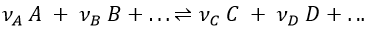Where A, B, C and D are chemical species and υI is the stoichiometric coefficient related to the species I. The reaction can be an elementary reaction or a complex reaction composed of several steps. The advancement of the reaction ζ can be followed by the variation of the amount of one species i.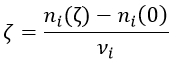We can choose any species involved in the reaction as the studied species. The speed of reaction is given by the derivative of the advancement of the reaction at a given temperature. As we want only one characteristic speed of reaction, we divide the derivative by the stoichiometric coefficient of the studied species. Also note that products are formed while reactants are consumed. To have a positive speed, a minus sign is added if the studied species is a reactant.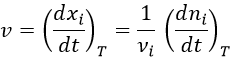Instead of the amount of particles ni, their concentration is followed. Note that the volume is thus part of the equation but it is generally constant.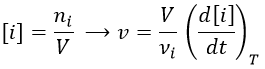Instead of the concentration, we can use the partial pressure for gases.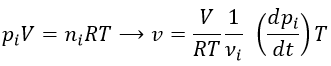Experimentally, we will thus measure the concentration or the pressure of one species over the time. The concentration of a product increases over time while the concentration of a reactant decreases. The speed is defined positive and a minus sign must thus be placed before the derivative of the concentration of a reaction.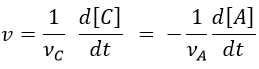Yet, the speed of the reaction depends on the time: while approaching the end of the reaction, there is less reactant in the solution and the probability that the molecules of reactant are in contact so that they can react decreases. We want thus a measure that is independent of the time and characteristic of the reaction. On a graph of the concentration versus the time, the speed is proportional to plus or minus the slope of the curve. Obviously the slope changes over the time.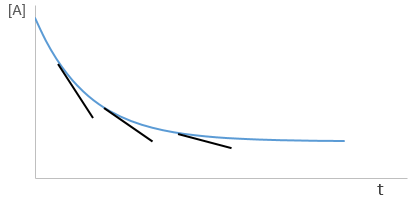Reactions can be subdivided as a function of the order of reaction.

Reaction of first order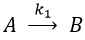It is for instance a reaction of decomposition or a catalysed reaction. k1 is the constant of equilibrium, with 1 as index to suggest the order of reaction. A is one reactant and B is one product of the reaction. The instantaneous speed is directly proportional to the instantaneous concentration.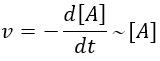The coefficient of proportionality is the constant of speed k1.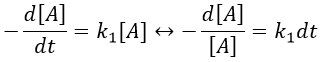The units of k1 are s-1: when we look at the equation, [A] and d[A] have the same units –mol- and dt is in s. Each side of the equation has to have the same units so k1 is in s-1. The resolution of the equation is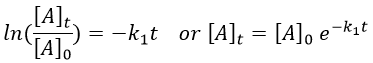with [A]0 and [A]t respectively the initial concentration of the reactant  and its concentration at time t. The concentration decreases exponentially over the time but we can plot the logarithm of the concentration ln[A] as a function of the time to find a straight line the slope of which is –k1.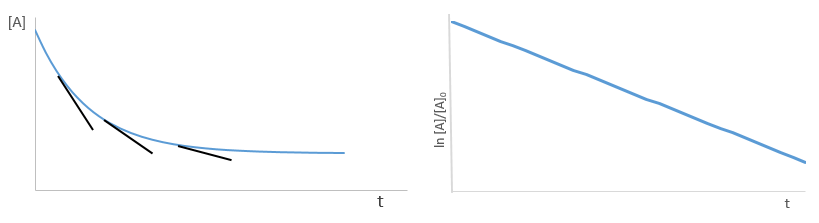If instead of measuring the evolution of the concentration of the reactant we measure the evolution of the concentration of the product B, we know that both concentrations are related. The amount of B that is formed is equal to the consumed amount of A, i.e. the initial amount of A minus the amount of A that is still in solution. If there was no product is solution at the beginning of the reaction, then our final concentration of product will be the initial concentration of the reactant.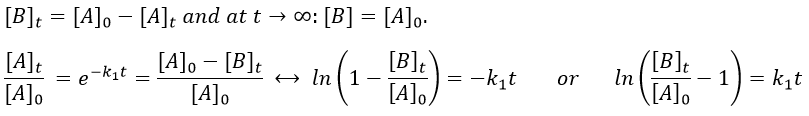We can thus also find a straight line the slope of which is k1.

A time of half-life, i.e. the time required for the consumption of half of the reactant A, can be determined. It is not equal to the half of the time required to consume all the reactant.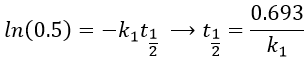This time is independent of the initial concentration. After t1/2, the concentration of reactant is divided by 2. After 2 t1/2, the concentration is divided by 4. After 3 t1/2, by 8, etc. The measurement of those times we can confirm the value of k1 that was found by the slope of ln [A].

The concentration can be followed by a measure of the absorbance of the solution, of its pH, conductivity or optical activity.

Reaction of order 2

If we consider only one reactant and we don’t find the previous relations, the reaction can be of a higher order than the order 1. Two identical molecule can merge to form one new product, by condensation for example (amino acids).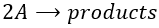In this case, the speed of reaction depends on the square of the concentration of the reactant.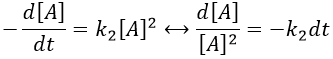Note that this constant of reaction k2 has not the same units that the constant of reaction k1 of reactions of order 1. In d[A]/dt=k2[A]2, we have the square of a concentration at one side of the equation and a concentration at the other side (with a variation of time). The units of k2 are mol-1s-1. If we consider a gas, we will follow the pressure pA of the reactant.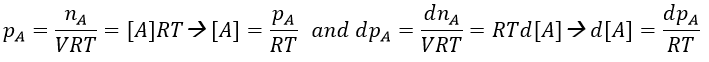As a result,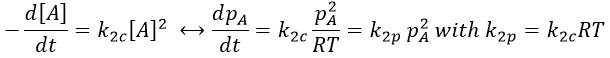In a general way, we have kmp=kmc(RT)m-1 with m the order of the reaction.

The solution of the equation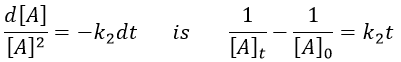If we plot the logarithm of the concentration, we won’t find a straight line this time. To obtain one, we have to plot 1/[A]. The slope of the line is the constant of speed of the reaction.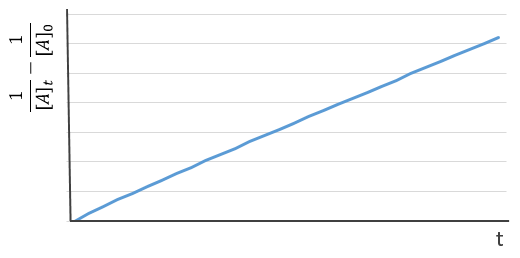The half-life time is obtained from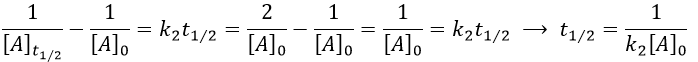This time, t1/2 depends on the initial concentration of the reactant. The half of the concentration is consumed over t1/2. The time to consume half of the remaining concentration [A]0’ (i.e. to consume the next [A]0/4) is not the same time but twice as long because the initial concentration is now [A]0’ and is the half of the initial concentration [A]0.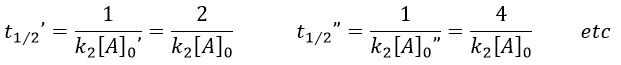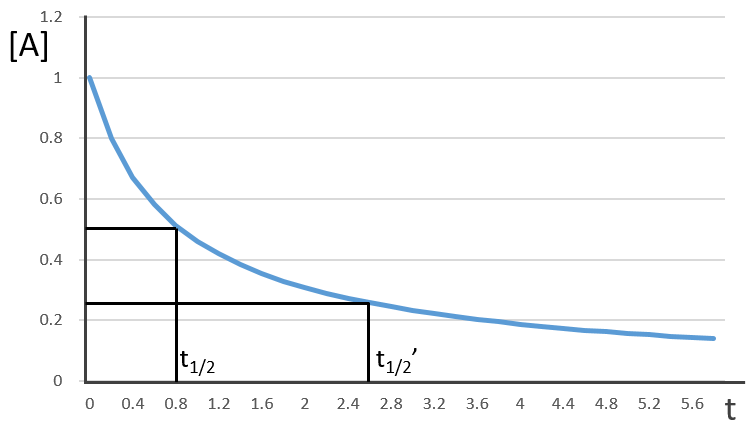The half-life time becomes longer and longer with the advancement of the reaction.

Reactions of order 2 can also involve two different reactants.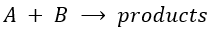A and B are consumed at the same rate but the concentrations can be different if there was an excess of one of the reactants.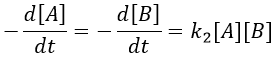At a given time, a concentration x of A and of B has been consumed by the process. We can thus replace the concentration at that time by the difference between the initial concentration and x.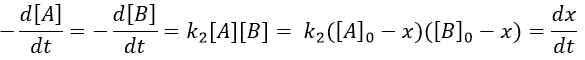From two parameters we have now only one parameter, x and the equation can be solved.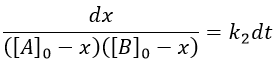We can integrate the equation over the time of the reaction (from t=0 to t and from x=0 to x).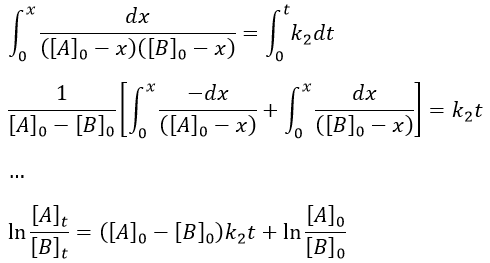If [A]0=[B]0, then the ratio of concentrations [A]/[B] always remains constant but we won’t be able to determine k. The trick is to put one reactant in large excess and to follow the concentration of the second reactant. If one reactant is put in excess, then the expression for the concentration is more complicated. But if we put an excess large enough to consider the concentration of the other reactant as negligible, then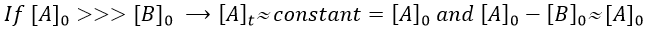Then we obtain a pseudo-order 1 reaction.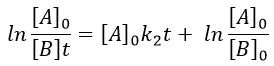The second logarithm can be moved in the first: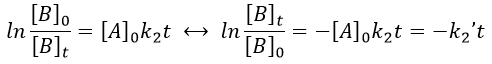with k2’=[A]0k2 a kinetic constant of (pseudo)order 1. As we can find k2’, we know k2=k2’/[A]0.

The bimolecular nucleophile substitution (SN2) is a reaction the mechanism of which was found by regular kinetics studies.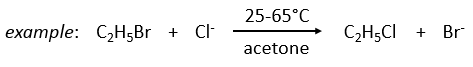On the other hand the SN1 reaction showed extravagant results during the kinetics studies: the speed of the reaction was independent of the initial concentration of one reactant.

Reactions of order 0

In this kind of reaction, the speed of reaction does not depend on the concentration of one or more reactants.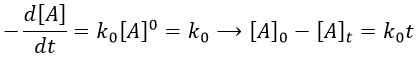It is typically the case when the reaction is made through several steps. We also find order 0 if there is a limiting factor such as the surface of a catalyst. The speed of reaction is then constant over the time. If we take the case of one SN1, the reaction involves 2 reactants but does not show the expected order 2 for the reaction.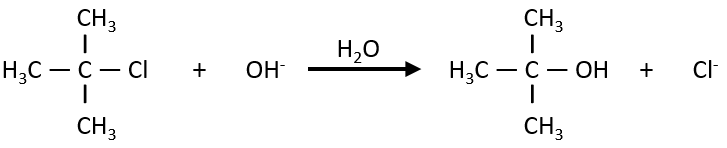The reaction shows a kinetics of order 1 with regards to the chlorobutane and order 0 with regards to the hydroxide. The reason is that the reaction is made in two steps and the determining step is the first step in which OH takes no part. The first step involves the formation of a carbocation from the chlorobutane, i.e. a reaction of order 1, and then a reaction of the second order that is much faster than the first reaction.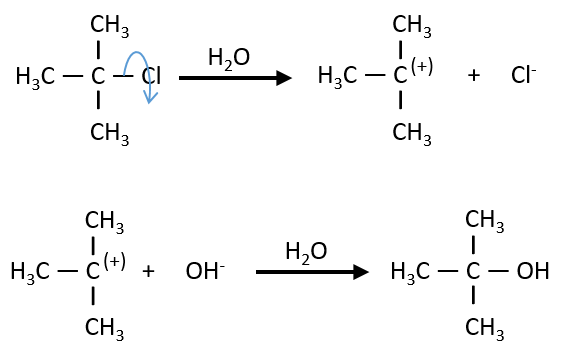As the second reaction is much faster than the first, it is not this reaction that limits the global speed of reaction and we detect experimentally only the first reaction that does not include OH. We can verify the order 0 by putting a large excess of the alkane in the solution and measure the evolution of OH. It will decreases proportionally to the time and confirm the order 0.

An example of the utility of the kinetics is the synthesis of glycerol starting from epichlorohydrin made by Ernest Solvay.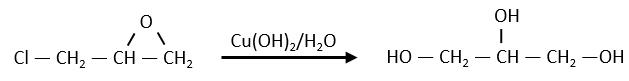The glycerol and the epichlorohydrin are both transparent but once the reactant were put in the reacting tank and heated the system, the solution turned yellow-brown. They though that they overheated the system but it was still the same with a lower heating. A PhD in kinetics was consulted on the subject. He found out that the reaction is of order 0 with regards to the epichlorohydrin, meaning that the reaction involves several steps. If they heated up the reaction, they found an order 1 reaction. In fact, there is a determining step before the reaction: the dissolution of the epichlorohydrin into water.

As we have seen, a reaction can have a different order depending on the observed reactant. The global order n of the reaction is the sum of the orders with regards to each reactant.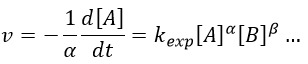Where α, β, … are the order with regards to A, B, … and n=α+β+… is the order of reaction. There are several methods to determine experimentally the orders of a reaction with regards to the reactants

Method of integration

This method uses the relation between the evolution of the concentration of one given species over the time to determine the order of reaction with regards to this species: we verify if we can obtain a straight line on the graph of ln[A] vs time (order 1), of 1/[A] vs time (order 2) or of [A] vs time (order 0).

Method of half-life time fraction

We use here the properties of the half-life time if the reaction speed only depends on one of the reactants (the others are put in excess). We vary the initial concentration of the studied reactant.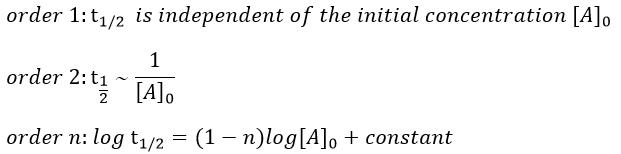Method of isolement

All the reactants except one are in excess. We measure the order of the reaction with regards to this reactant.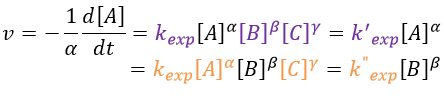We proceed the same way with a different reactant (no excess of concentration) to determine the pseudo-orders of reaction with regards to each analysed reactant and we combine the result to find the order of reaction.

Method of the initial speeds

As usual we measure the evolution of the concentration as a function of the time. The tangent of the curve at t=0 gives the initial speed v0 (-v0 to be accurate). One reactant is not put in excess and is measured.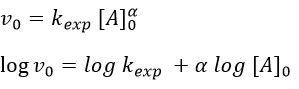The experiment is repeated several times (it is quick because we only look at the beginning of the reaction) for several initial concentrations. This way we can determine kexp and α. This method is not very accurate because we measure a tangent at t=0.

When we study the kinetics of a reaction, we must always at least use the method of integration and of initial speeds and obtain concordant results. If it is not the case, the supposed mechanism is not correct.